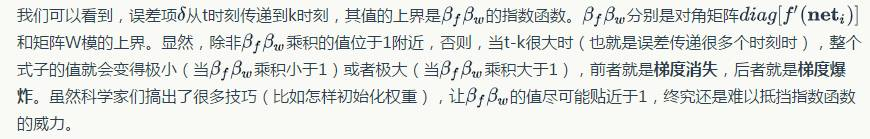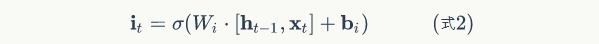#0
1
01. 云栖社区>
2. 博客>
3. 正文

## 零基础入门深度学习(6) - 长短时记忆网络(LSTM)

lhyxcxy 2017-01-24 17:33:00 浏览7235LSTM的关键，就是怎样控制长期状态c。在这里，LSTM的思路是使用三个控制开关。第一个开关，负责控制继续保存长期状态c；第二个开关，负责控制把即时状态输入到长期状态c；第三个开关，负责控制是否把长期状态c作为当前的LSTM的输出。三个开关的作用如下图所示：LSTM最终的输出，是由输出门和单元状态共同确定的：LSTM训练算法框架

LSTM的训练算法仍然是反向传播算法，对于这个算法，我们已经非常熟悉了。主要有下面三个步骤：```    class SigmoidActivator(object):
def forward(self, weighted_input):
return 1.0 / (1.0 + np.exp(-weighted_input))
def backward(self, output):
return output * (1 - output)
class TanhActivator(object):
def forward(self, weighted_input):
return 2.0 / (1.0 + np.exp(-2 * weighted_input)) - 1.0
def backward(self, output):
return 1 - output * output```

LSTM初始化

```    class LstmLayer(object):
def __init__(self, input_width, state_width,
learning_rate):
self.input_width = input_width
self.state_width = state_width
self.learning_rate = learning_rate
# 门的激活函数
self.gate_activator = SigmoidActivator()
# 输出的激活函数
self.output_activator = TanhActivator()
# 当前时刻初始化为t0
self.times = 0
# 各个时刻的单元状态向量c
self.c_list = self.init_state_vec()
# 各个时刻的输出向量h
self.h_list = self.init_state_vec()
# 各个时刻的遗忘门f
self.f_list = self.init_state_vec()
# 各个时刻的输入门i
self.i_list = self.init_state_vec()
# 各个时刻的输出门o
self.o_list = self.init_state_vec()
# 各个时刻的即时状态c~
self.ct_list = self.init_state_vec()
# 遗忘门权重矩阵Wfh, Wfx, 偏置项bf
self.Wfh, self.Wfx, self.bf = (
self.init_weight_mat())
# 输入门权重矩阵Wfh, Wfx, 偏置项bf
self.Wih, self.Wix, self.bi = (
self.init_weight_mat())
# 输出门权重矩阵Wfh, Wfx, 偏置项bf
self.Woh, self.Wox, self.bo = (
self.init_weight_mat())
# 单元状态权重矩阵Wfh, Wfx, 偏置项bf
self.Wch, self.Wcx, self.bc = (
self.init_weight_mat())
def init_state_vec(self):
'''
初始化保存状态的向量
'''
state_vec_list = []
state_vec_list.append(np.zeros(
(self.state_width, 1)))
return state_vec_list
def init_weight_mat(self):
'''
初始化权重矩阵
'''
Wh = np.random.uniform(-1e-4, 1e-4,
(self.state_width, self.state_width))
Wx = np.random.uniform(-1e-4, 1e-4,
(self.state_width, self.input_width))
b = np.zeros((self.state_width, 1))
return Wh, Wx, b```

forward方法实现了LSTM的前向计算：

```def forward(self, x):
'''
根据式1-式6进行前向计算
'''
self.times += 1
# 遗忘门
fg = self.calc_gate(x, self.Wfx, self.Wfh,
self.bf, self.gate_activator)
self.f_list.append(fg)
# 输入门
ig = self.calc_gate(x, self.Wix, self.Wih,
self.bi, self.gate_activator)
self.i_list.append(ig)
# 输出门
og = self.calc_gate(x, self.Wox, self.Woh,
self.bo, self.gate_activator)
self.o_list.append(og)
# 即时状态
ct = self.calc_gate(x, self.Wcx, self.Wch,
self.bc, self.output_activator)
self.ct_list.append(ct)
# 单元状态
c = fg * self.c_list[self.times - 1] + ig * ct
self.c_list.append(c)
# 输出
h = og * self.output_activator.forward(c)
self.h_list.append(h)
def calc_gate(self, x, Wx, Wh, b, activator):
'''
计算门
'''
h = self.h_list[self.times - 1] # 上次的LSTM输出
net = np.dot(Wh, h) + np.dot(Wx, x) + b
gate = activator.forward(net)
return gate```

backward方法实现了LSTM的反向传播算法。需要注意的是，与backword相关的内部状态变量是在调用backward方法之后才初始化的。这种延迟初始化的一个好处是，如果LSTM只是用来推理，那么就不需要初始化这些变量，节省了很多内存。

```        def backward(self, x, delta_h, activator):
'''
实现LSTM训练算法
'''
self.calc_delta(delta_h, activator)

```        def calc_delta(self, delta_h, activator):
# 初始化各个时刻的误差项
self.delta_h_list = self.init_delta()  # 输出误差项
self.delta_o_list = self.init_delta()  # 输出门误差项
self.delta_i_list = self.init_delta()  # 输入门误差项
self.delta_f_list = self.init_delta()  # 遗忘门误差项
self.delta_ct_list = self.init_delta() # 即时输出误差项
# 保存从上一层传递下来的当前时刻的误差项
self.delta_h_list[-1] = delta_h
# 迭代计算每个时刻的误差项
for k in range(self.times, 0, -1):
self.calc_delta_k(k)
def init_delta(self):
'''
初始化误差项
'''
delta_list = []
for i in range(self.times + 1):
delta_list.append(np.zeros(
(self.state_width, 1)))
return delta_list
def calc_delta_k(self, k):
'''
根据k时刻的delta_h，计算k时刻的delta_f、
delta_i、delta_o、delta_ct，以及k-1时刻的delta_h
'''
# 获得k时刻前向计算的值
ig = self.i_list[k]
og = self.o_list[k]
fg = self.f_list[k]
ct = self.ct_list[k]
c = self.c_list[k]
c_prev = self.c_list[k-1]
tanh_c = self.output_activator.forward(c)
delta_k = self.delta_h_list[k]
# 根据式9计算delta_o
delta_o = (delta_k * tanh_c *
self.gate_activator.backward(og))
delta_f = (delta_k * og *
(1 - tanh_c * tanh_c) * c_prev *
self.gate_activator.backward(fg))
delta_i = (delta_k * og *
(1 - tanh_c * tanh_c) * ct *
self.gate_activator.backward(ig))
delta_ct = (delta_k * og *
(1 - tanh_c * tanh_c) * ig *
self.output_activator.backward(ct))
delta_h_prev = (
np.dot(delta_o.transpose(), self.Woh) +
np.dot(delta_i.transpose(), self.Wih) +
np.dot(delta_f.transpose(), self.Wfh) +
np.dot(delta_ct.transpose(), self.Wch)
).transpose()
# 保存全部delta值
self.delta_h_list[k-1] = delta_h_prev
self.delta_f_list[k] = delta_f
self.delta_i_list[k] = delta_i
self.delta_o_list[k] = delta_o
self.delta_ct_list[k] = delta_ct```

```        def calc_gradient(self, x):
# 初始化遗忘门权重梯度矩阵和偏置项
# 初始化输入门权重梯度矩阵和偏置项
# 初始化输出门权重梯度矩阵和偏置项
# 初始化单元状态权重梯度矩阵和偏置项
# 计算对上一次输出h的权重梯度
for t in range(self.times, 0, -1):
# 计算各个时刻的梯度
# 实际梯度是各时刻梯度之和
print '-----%d-----' % t
# 计算对本次输入x的权重梯度
xt = x.transpose()
'''
初始化权重矩阵
'''
self.state_width))
self.input_width))
'''
计算每个时刻t权重的梯度
'''
h_prev = self.h_list[t-1].transpose()

### 梯度下降算法的实现

```        def update(self):
'''
按照梯度下降，更新权重
'''

```        def reset_state(self):
# 当前时刻初始化为t0
self.times = 0
# 各个时刻的单元状态向量c
self.c_list = self.init_state_vec()
# 各个时刻的输出向量h
self.h_list = self.init_state_vec()
# 各个时刻的遗忘门f
self.f_list = self.init_state_vec()
# 各个时刻的输入门i
self.i_list = self.init_state_vec()
# 各个时刻的输出门o
self.o_list = self.init_state_vec()
# 各个时刻的即时状态c~
self.ct_list = self.init_state_vec()```

```    def data_set():
x = [np.array([, , ]),
np.array([, , ])]
d = np.array([, ])
return x, d
'''
梯度检查
'''
# 设计一个误差函数，取所有节点输出项之和
error_function = lambda o: o.sum()
lstm = LstmLayer(3, 2, 1e-3)
# 计算forward值
x, d = data_set()
lstm.forward(x)
lstm.forward(x)
# 求取sensitivity map
sensitivity_array = np.ones(lstm.h_list[-1].shape,
dtype=np.float64)
# 计算梯度
lstm.backward(x, sensitivity_array, IdentityActivator())
# 检查梯度
epsilon = 10e-4
for i in range(lstm.Wfh.shape):
for j in range(lstm.Wfh.shape):
lstm.Wfh[i,j] += epsilon
lstm.reset_state()
lstm.forward(x)
lstm.forward(x)
err1 = error_function(lstm.h_list[-1])
lstm.Wfh[i,j] -= 2*epsilon
lstm.reset_state()
lstm.forward(x)
lstm.forward(x)
err2 = error_function(lstm.h_list[-1])
expect_grad = (err1 - err2) / (2 * epsilon)
lstm.Wfh[i,j] += epsilon
print 'weights(%d,%d): expected - actural %.4e - %.4e' % (
return lstm```GRU

GRU对LSTM做了两个大改动：

1. 将输入门、遗忘门、输出门变为两个门：更新门（Update Gate）Zt和重置门（Reset Gate）rt。

2. 将单元状态与输出合并为一个状态：h。

GRU的前向计算公式为：GRU的训练算法比LSTM简单一些，留给读者自行推导，本文就不再赘述了。

lhyxcxy
+ 关注